Rabbits

Michael breeding rabbits. His 7 rabbits will eat 98 feed rations for 7 days. How many equal portions of feed should be eat 5 rabbits in 6 days?

Result

x =  60

Solution:Leave us a comment of this math problem and its solution (i.e. if it is still somewhat unclear...):Be the first to comment!Next similar math problems:

1. DonutsFind how many donuts each student will receive if you share 126 donuts in a ratio of 1:5:8
2. RunnersFor three runners (on the first to third place) is prepared 30 chocolate that they be distributed in the ratio of 3 : 2 : 1 How much chocolate will get everyone?
3. Liquid soap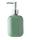Three-member family enough liter of liquid soap for 20 days. After how many days the amount consumed when at the holidays come to us two cousins.
4. Chocolate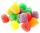I eat 24 chocolate in 10 days. How many chocolate I eat in 15 days at the same pace?
5. AgesJohn, Teresa, Daniel and Paul have summary 56 years. Their ages are in a ratio of 1:2:5:6. Determine how many years have each of them.
6. BuildingAt the building, we divided 240 boards into two piles in a 5: 3 ratio. How many were fewer boards in the lower pile?
7. Greenhouses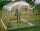Two workers would make installation of greenhouses for 54 hours. For how many hours does the installation 9 workers?
8. Two machinesPerformances of two machines are in a ratio of 7:12. A machine with less power produced 406 pieces of products per shift. a) How many pieces produced per shift second machine? b) How many pieces produced two machines together for five shifts?
9. BicycleThe bicycle pedal gear has 36 teeth, the rear gear wheel has 10 teeth. How many times turns rear wheel, when pedal wheel turns 120x?
10. Train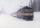The train passes part of the line for 95 minutes at speed 75 km/h. What speed would have to go in order to shorten the driving time of 20 minutes?
11. RatiosReduce the numbers: 50 in a 1:2 ratio 111 at a ratio of 2:3 70 at 10:50 560 at a ratio of 3:8
12. Guppies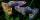Audrey has some guppies in a fish tank. The ration of the oranges guppies to silver guppies is 3:5. She has 12y oranges guppies. Write the number of silver guppies she has in terms of y
13. Moneys in triple ratioMilan, John and Lili have a total 344 euros. Their amounts are in the ratio 1:2:5. Determine how much each of them has?
14. PeppersIn the box are yellow (a), green (b) and red (c) peppers. Their amount is in a ratio 2:4:1 . Most are yellow peppers and green the least. Calculate the number of peppers each type if the total number of peppers is 70.
15. Divide moneyDivide 1200 USD at a ratio of 1:2:3:4:5:6:9:10
16. Addition of Roman numbersAdded together and write as decimal number: LXVII + MLXIV
17. Reciprocal equation 3Solve reciprocal equation: 1/2 + 2/3=1/x